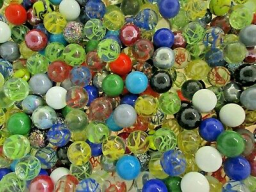# Bag of marbles

In a bag full of marbles, 1/4 of these marbles are green, 1/8 are blue, 1/2 are yellow, and the remaining 26 are white. How many marbles are blue?

b =  26

### Step-by-step explanation:Did you find an error or inaccuracy? Feel free to write us. Thank you!

Tips for related online calculators
Need help calculating sum, simplifying, or multiplying fractions? Try our fraction calculator.
Do you have a linear equation or system of equations and looking for its solution? Or do you have a quadratic equation?